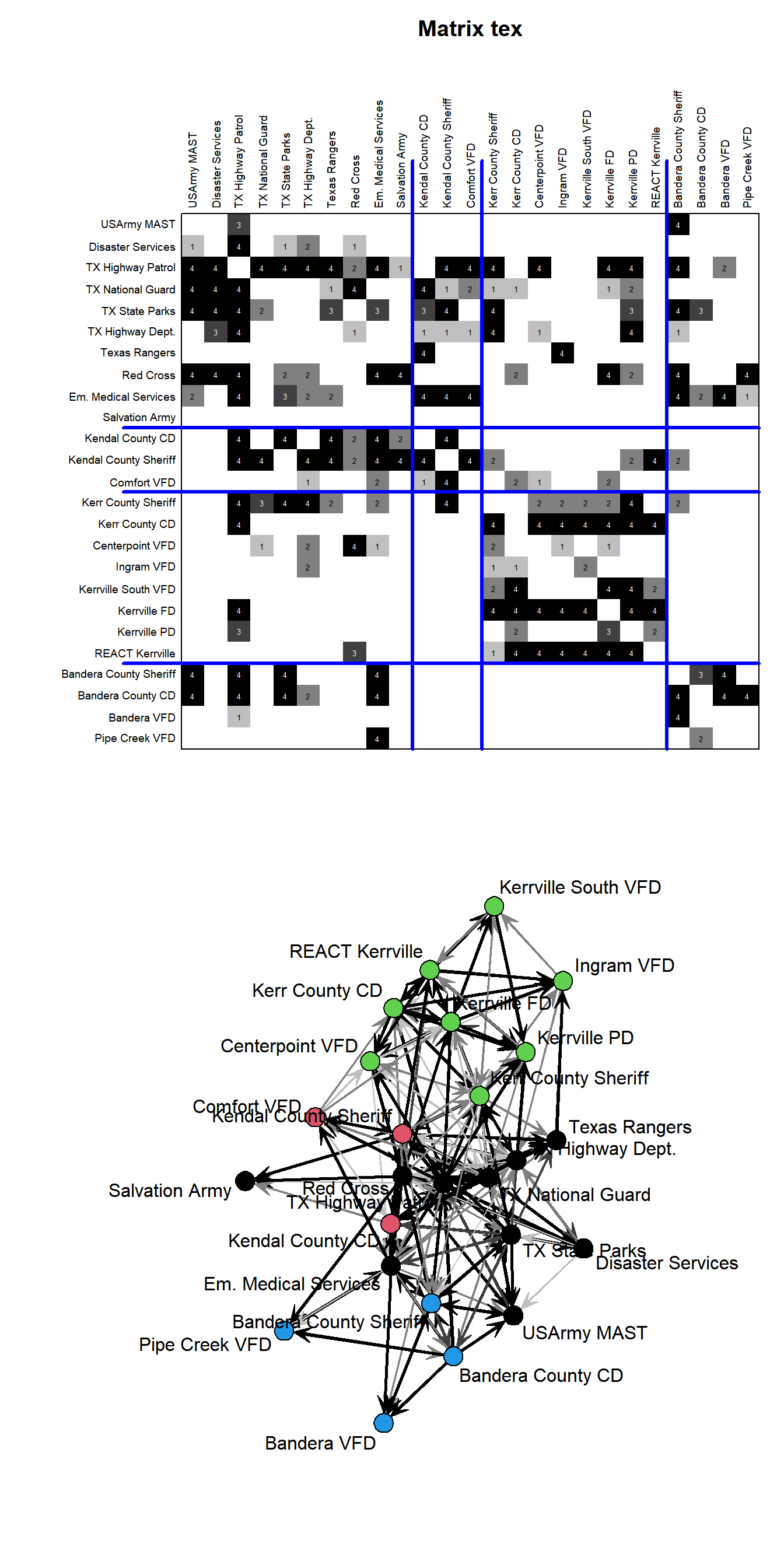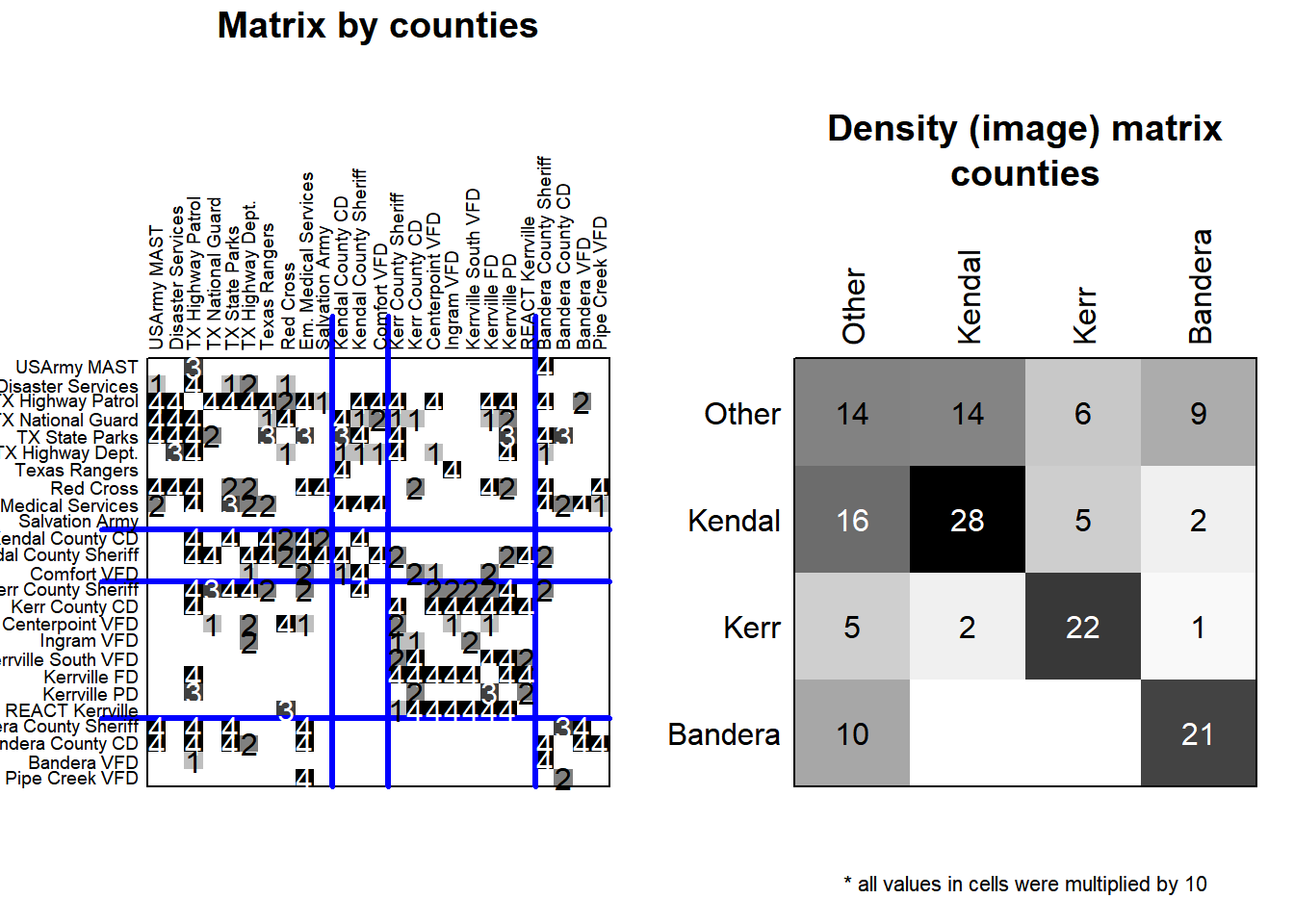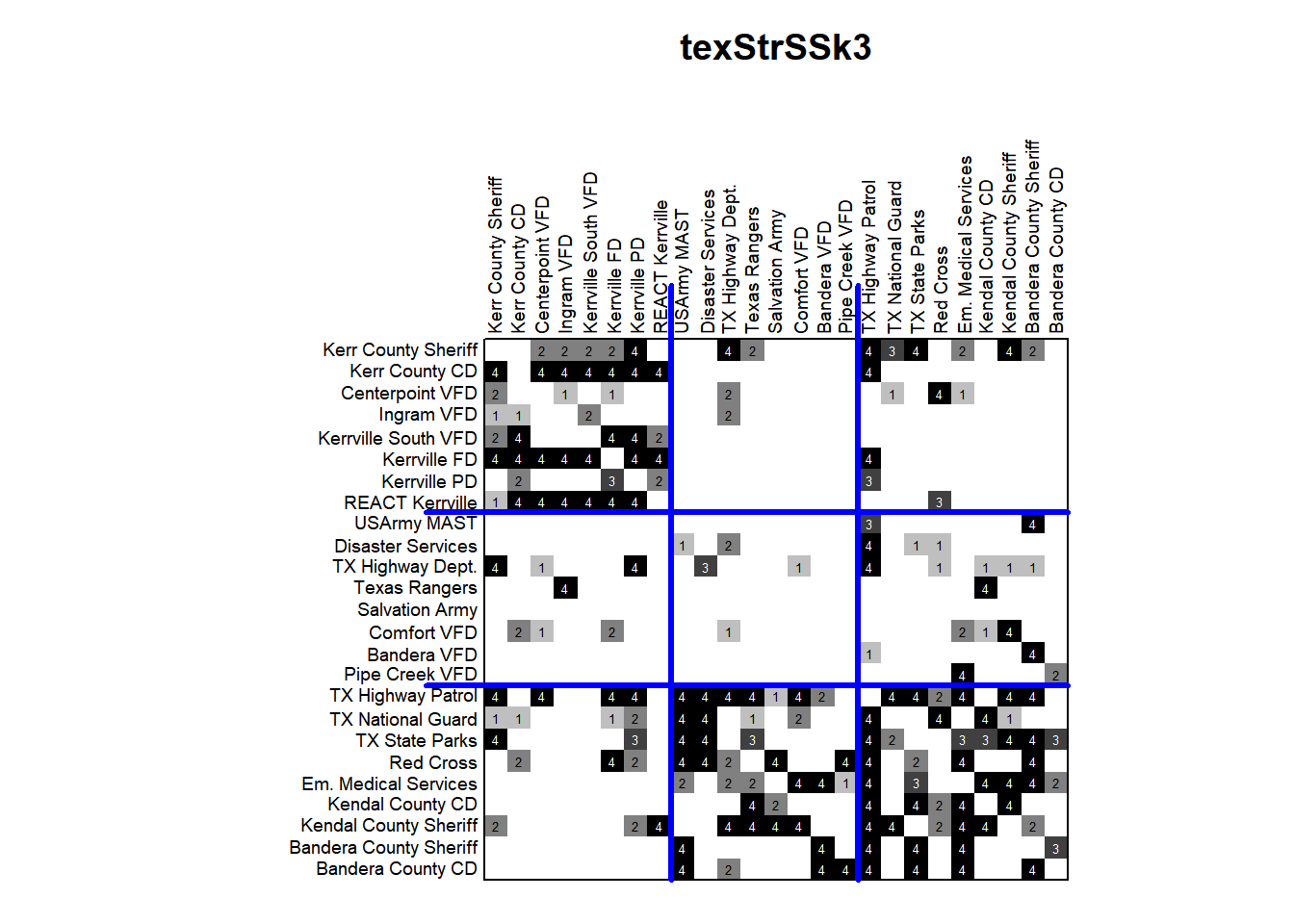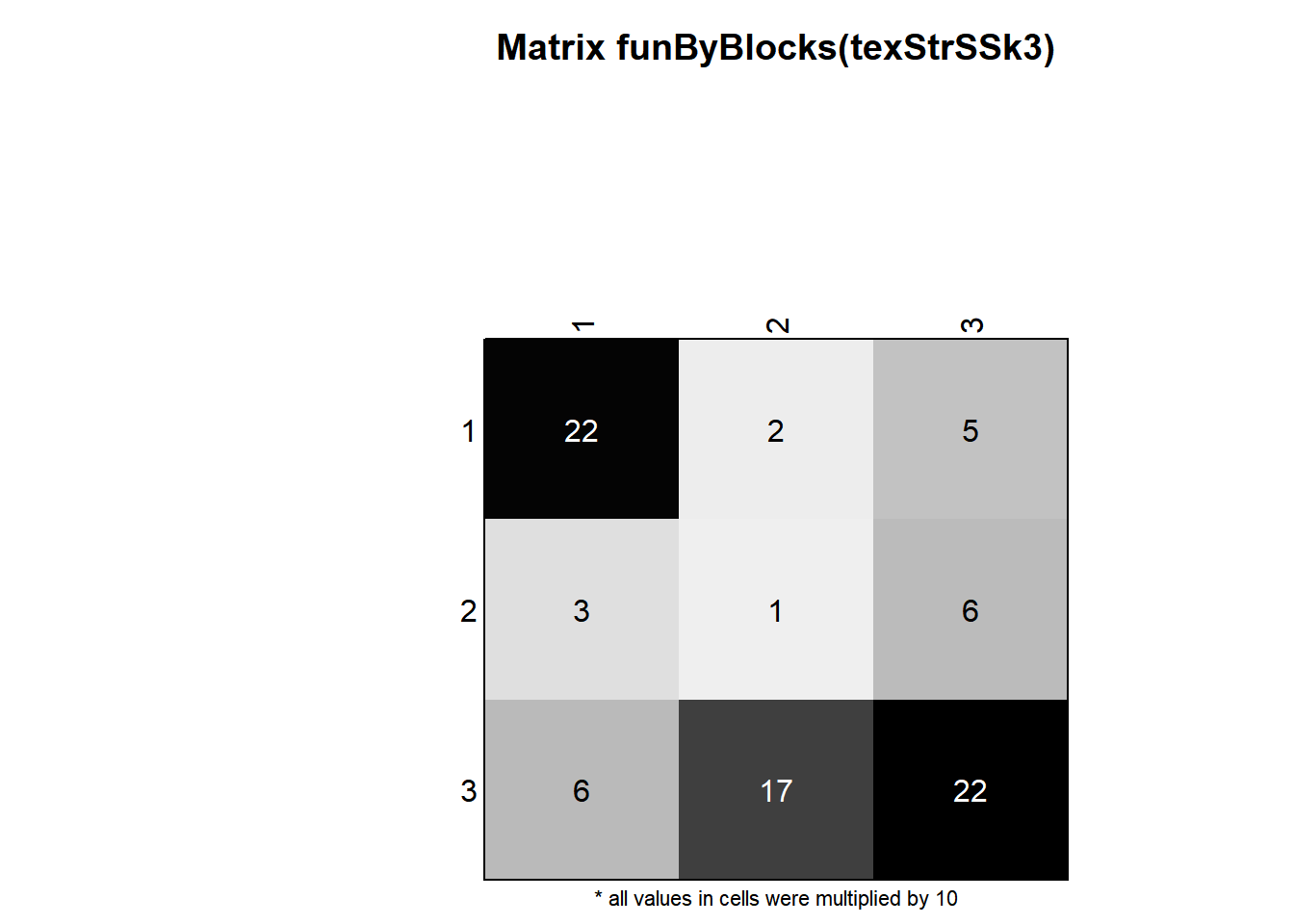# The data

## Description

The network represents communication between organizations involved in search and rescue operations in three Texas counties (Kerr, Kendal and Bandera) after 1978 flood.

Drabek et al. (1981) provided seven case studies of emergent multi-organizational networks in the context of search and rescue (SAR) activities. Networks of interaction frequency are reported, along with several organizational attributes.

Our network was receded so that higher value means more frequent communication -> 1->4, 2->3, 3->2, 4->1 The tie values now mean:

• 0 - “no communication”
• 1 - “about once a day or less”
• 2 - “every few hours”
• 3 - “about once an hour”
• 4 - “continuously”

For more see https://rdrr.io/cran/network/man/emon.html.

First we load the blockmodeling and sna packages and the data (available on workshop web page).

``````library(blockmodeling)
library(sna)

tex<-loadmatrix("texas.net") #File is in Pajek matrix format.
texCounty<-factor(texCounty,labels=c("Other","Kendal","Kerr","Bandera"))``````

## Ploting a partitioned matrix

Then we plot the network and partition by counties in matrix and graph form. We use the `plotMat` function with paramters `M` (matrix) and `clu` (partition).

``````par(mfrow=c(2,1))
plotMat(tex, clu=texCounty)
gplot1( tex/2,vertex.col=texCounty)``````## Ploting an image matrix

Based on network and a partition, we can get an image matrix (means by blocks) with `funByBlocks`.

``````par(mfrow=c(1,2))
plotMat(tex, clu=texCounty,mar=c(1,4,7,1),x.axis.val.pos = 1.02, y.axis.val.pos = -0.02, main="Matrix by counties",title.line=6,cex.val = 1)

tmpDensIM<-funByBlocks(tex,clu=texCounty,na.rm=TRUE)
plotMat(tmpDensIM,title.line=2,main="Density (image) matrix\ncounties",mar=c(1,4,7,1),x.axis.val.pos = 1.03, y.axis.val.pos = -0.03)````````par(mfrow=c(1,1))``

# Generalized blockmodeling

For finding a partition using generalized blockmodeling, we use `optRandomParC` function (also on slides 33-26). The function searches for the best partition of a network (matrix) into a specified numner of clusters according to a selected equivalence. It’s main arguments are:

• `M` – The network in (dense) matrix format. An array for multirelational network
• `k` – the number of clusters. If data contain more sets, this is a vector (advanced).
• `approaches` – one of `"bin"` (binary), `"val"` (valued) or `"hom"` (homogeneity) blockmodeling.
• `blocks` – allowed block types
• `rep` – the number of random starting partitions
• `nCores` – For multicore computers. Defaults to 1. 0 means automatic (number of available cores – 1).

`blocks` parameter specifies the equivalences as:

• A character vector of allowed block types or a list of such vectors for multirelational networks.
• A pre-specified blockmodel: An array with four dimensions. The third and the fourth represent the clusters (for rows and columns). The first is as long as the maximum number of allows block types for a given block. If some block has less possible block types, the empty slots should have values NA. The second dimension is the number of relations (can be omitted for single-relational networks or set to 1). The values in the array should be the ones from the next slide.

Block types (possible values in vector or array supplied to `blocks`) are:

• `"nul"` - null or empty block
• `"com"` - complete block
• `"rdo"`, `"cdo"` - row and column-dominant blocks (binary and valued approach only)
• `"reg"` - (f-)regular block
• `"rre"`, `"cre"` - row and column-(f-)regular blocks
• `"rfn"`, `"cfn"` - row and column-dominant blocks (binary and valued approach only)
• `"den"` - density block (binary approach only)
• `"avg"` - average block (valued approach only)
• `"dnc"` - do not care block - the error is always zero The ordering is important, since if several block types have identical error, the first on the list is selected.

## Homogeneity blockmodeling

We will start with homogeneity blockmodeling, as the least preparations are needed to use it.

For homogeneity blockmodeling we set `approaches="hom"`. Sum of squares blockmodeling is used by default, but we can also explicitly demand it with `homFun="ss"`. For absolute deviations blockmodeling, we would have to specify `homFun="ad"`.

### Structural equivalence

The equivalence is specified via `blocks` argument. For structural equivalence, null (`"nul"`) and complete (`"com"`) block types are allowed, but as for homogeneity blockmodeling, the complete block is a special case of the null block, only complete block can be specified (`blocks=c("com")`). In the example below, we specify:

• `M=tex` - the data is in matrix `tex`.
• `k=3` - we want partitions into 3 clusters.
• `rep=200` - we want 200 repetitions, that is start with 300 random starting partitions.
• `blocks=c("com")` - structural equivalence (se above)
• `approaches="hom",homFun="ss"`- homogeneity sum of squares blockmodeling
• `nCores=0` - the number of cores is set to “number of available cores” - 1.

Then we use method `plot` (which calles `plotMat`) to plot the results.

``````set.seed(2021)
``## Loading required namespace: doParallel``
``## Loading required namespace: doRNG``
``````##
##
## Optimization of all partitions completed
## 1 solution(s) with minimal error = 1072.744 found.``````

The function has found 1 partition with the minimal value of the criterion function = 1072.744.

Then we print the resoult and plot the matrix.

``texStrSSk3``
``````## Network size: 25
##
## Approachs (paramter): hom-ss
## Blocks (paramter)
## com
##
## Sizes of clusters:
## 1 2 3
## 8 8 9
##
## Error: 1072.744``````
``plot(texStrSSk3)``We see we got 3 clusters of approximately equal size.

Now we can plot na image matrix.

``plotMat(funByBlocks(texStrSSk3))``We can make things nicer by giving the names to clusters. Here we also use the argument `orderClu` of function `funByBlocks` to order the clusters by decreasing average in-degrees, so that the order is not random.

``````## Making a nice image matrix